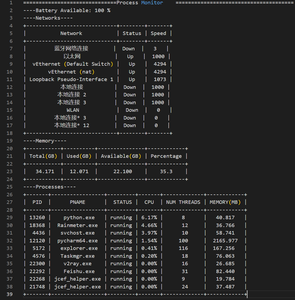# How to Make a Process Monitor in Python?

• Difficulty Level : Expert
• Last Updated : 18 Aug, 2022

A process monitor is a tool that displays the system information like processes, memory, network, and other stuff. There are plenty of tools available, but we can make our own process monitor using Python. In Python, there is a module called psutil that we can use to grab various information about our system

## Modules Needed

• psutil: Type the below command in the terminal to install this module.
`python3 -m pip install psutil `
• Prettytable: To print the data on console, we can use a formatter module PrettyTable:
`python3 -m pip install prettytable`

## Using psutil

psutil provides lots of features to monitor the system. We will see some of them in brief:

• First, we need to import psutil:
`import psutil`
• List the process ids:
`psutil.pids()  # [1,2,.....4352]`
• Fetch process information:
```process_id = 1
psutil.Process(process_id)
# psutil.Process(pid=1, name='systemd', status='sleeping', started='19:49:25')```
• We can access various keys of this process:
```process = psutil.Process(process_id)
process.name()
process.status()```
• Accessing battery status:
```psutil.sensors_battery()
psutil.sensors_battery().percent```
• Accessing Network Interfaces:
```psutil.net_if_stats()
psutil.net_if_stats()['wlo1'].isup    # True```
• We can also check the memory:
```psutil.virtual_memory()
psutil.virtual_memory().total    # 8180498432 (In Bytes)
psutil.virtual_memory().used    # 2155720704
psutil.virtual_memory().available   # 5563060224```

Now that we know some basic features, we can implement the process monitor. Create a new python file and add the following code in it. The code below works on Linux distributions. For other operating systems, some functions may slightly differ.

Approach:

• Import the required packages.
• Clear the console using the call() function of the subprocess module. We can use the ‘clear’ or ‘cls’ command depending on OS.
• Fetch the battery information
• Fetch the network information and print it as PrettyTable
• Fetch the memory information
• Fetch the process information
• Create a delay. We have created a 1-second delay using time.sleep(1)
• Press CTRL+C to stop the program.

Below is the implementation:

## Python3

 `# Import the required libraries` `import` `psutil` `import` `time` `from` `subprocess ``import` `call` `from` `prettytable ``import` `PrettyTable`   `# Run an infinite loop to constantly monitor the system` `while` `True``:`   `    ``# Clear the screen using a bash command` `    ``call(``'clear'``)`   `    ``print``("``=``=``=``=``=``=``=``=``=``=``=``=``=``=``=``=``=``=``=``=``=``=``=``=``=``=``=``=``=``=``Process Monitor\` `    ``=``=``=``=``=``=``=``=``=``=``=``=``=``=``=``=``=``=``=``=``=``=``=``=``=``=``=``=``=``=``=``=``=``=``=``=``=``=``")`   `    ``# Fetch the battery information` `    ``battery ``=` `psutil.sensors_battery().percent` `    ``print``(``"----Battery Available: %d "` `%` `(battery,) ``+` `"%"``)`   `    ``# We have used PrettyTable to print the data on console.` `    ``# t = PrettyTable()` `    ``# t.add_row()`   `    ``# Fetch the Network information` `    ``print``(``"----Networks----"``)` `    ``table ``=` `PrettyTable([``'Network'``, ``'Status'``, ``'Speed'``])` `    ``for` `key ``in` `psutil.net_if_stats().keys():` `        ``name ``=` `key` `        ``up ``=` `"Up"` `if` `psutil.net_if_stats()[key].isup ``else` `"Down"` `        ``speed ``=` `psutil.net_if_stats()[key].speed` `        ``table.add_row([name, up, speed])` `    ``print``(table)`   `    ``# Fetch the memory information` `    ``print``(``"----Memory----"``)` `    ``memory_table ``=` `PrettyTable([``"Total(GB)"``, ``"Used(GB)"``,` `                                ``"Available(GB)"``, ``"Percentage"``])` `    ``vm ``=` `psutil.virtual_memory()` `    ``memory_table.add_row([` `        ``f``'{vm.total / 1e9:.3f}'``,` `        ``f``'{vm.used / 1e9:.3f}'``,` `        ``f``'{vm.available / 1e9:.3f}'``,` `        ``vm.percent` `    ``])` `    ``print``(memory_table)`   `    ``# Fetch the 10 processes from available processes that has the highest cpu usage` `    ``print``(``"----Processes----"``)` `    ``process_table ``=` `PrettyTable([``'PID'``, ``'PNAME'``, ``'STATUS'``,` `                                 ``'CPU'``, ``'NUM THREADS'``, ``'MEMORY(MB)'``])`   `    ``proc ``=` `[]` `    ``# get the pids from last which mostly are user processes` `    ``for` `pid ``in` `psutil.pids()[``-``200``:]:` `        ``try``:` `            ``p ``=` `psutil.Process(pid)` `            ``# trigger cpu_percent() the first time which leads to return of 0.0` `            ``p.cpu_percent()` `            ``proc.append(p)`   `        ``except` `Exception as e:` `            ``pass`   `    ``# sort by cpu_percent` `    ``top ``=` `{}` `    ``time.sleep(``0.1``)` `    ``for` `p ``in` `proc:` `        ``# trigger cpu_percent() the second time for measurement` `        ``top[p] ``=` `p.cpu_percent() ``/` `psutil.cpu_count()`   `    ``top_list ``=` `sorted``(top.items(), key``=``lambda` `x: x[``1``])` `    ``top10 ``=` `top_list[``-``10``:]` `    ``top10.reverse()`   `    ``for` `p, cpu_percent ``in` `top10:`   `        ``# While fetching the processes, some of the subprocesses may exit` `        ``# Hence we need to put this code in try-except block` `        ``try``:` `            ``# oneshot to improve info retrieve efficiency` `            ``with p.oneshot():` `                ``process_table.add_row([` `                    ``str``(p.pid),` `                    ``p.name(),` `                    ``p.status(),` `                    ``f``'{cpu_percent:.2f}'` `+` `"%"``,` `                    ``p.num_threads(),` `                    ``f``'{p.memory_info().rss / 1e6:.3f}'` `                ``])`   `        ``except` `Exception as e:` `            ``pass` `    ``print``(process_table)`   `    ``# Create a 1 second delay` `    ``time.sleep(``1``)`

Output:My Personal Notes arrow_drop_up
Recommended Articles
Page :